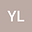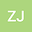loading page

Continuum of one-sign solutions of one-dimensional Minkowski-curvature problem with nonlinear boundary conditions
••Zhengqi Jing
Northwest Normal University
Author Profile## Abstract

In this work, we investigate the continuum of one-sign solutions of the nonlinear one-dimensional Minkowski-curvature equation $$-\big(u’/\sqrt{1-\kappa u’^2}\big)’=\lambda f(t,u),\ \ t\in(0,1)$$ with nonlinear boundary conditions $u(0)=\lambda g_1(u(0)), u(1)=\lambda g_2(u(1))$ by using unilateral global bifurcation techniques, where $\kappa>0$ is a constant, $\lambda>0$ is a parameter $g_1,g_2:[0,\infty)\to (0,\infty)$ are continuous functions and $f:[0,1]\times[-\frac{1}{\sqrt{\kappa}},\frac{1}{\sqrt{\kappa}}]\to\mathbb{R}$ is a continuous function. We prove the existence and multiplicity of one-sign solutions according to different asymptotic behaviors of nonlinearity near zero.

#### Peer review status:ACCEPTED

08 Jan 2021Submitted to Mathematical Methods in the Applied Sciences
08 Jan 2021Submission Checks Completed
08 Jan 2021Assigned to Editor
17 Jan 2021Reviewer(s) Assigned
16 Apr 2021Review(s) Completed, Editorial Evaluation Pending
19 Apr 2021Editorial Decision: Revise Major
13 May 20211st Revision Received
13 May 2021Submission Checks Completed
13 May 2021Assigned to Editor
13 May 2021Reviewer(s) Assigned
13 May 2021Review(s) Completed, Editorial Evaluation Pending
17 May 2021Editorial Decision: Accept